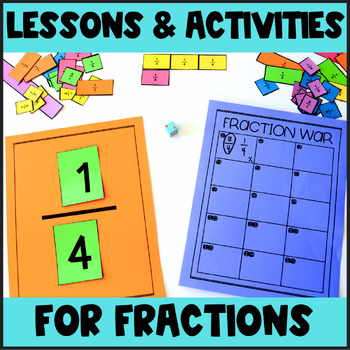# 3rd Grade Magic of Math Unit 5: FractionsGrade Levels
3rd
Subjects
Resource Type
Formats Included
• Zip
Pages
300+

#### Also included in

1. This BUNDLE contains all of our 3rd grade Magic of Math units (38 weeks of 3rd grade math instruction with EVERYTHING you need from minilessons, activities, interactive notebooking, games, word problems, and assessments!). To see a good description on each unit included, make sure you check out th
\$125.00
\$152.00
Save \$27.00

### Description

The Magic Of Math Unit 5 for THIRD GRADE focuses on:

Week 1: Intro to Fractions (parts of a group, parts of a whole, unit fractions, naming fractions, explaining fractional parts)

Week 2: Fractions on a Number Line, Fractions on Strip Diagrams, Sum of Unit Fractions, Composing and Decomposing Fractions

Week 3: Comparing Fractions (comparing fractions with the same denominator, comparing fractions with the same numerator, comparing fractions)

Week 4: Equivalent Fractions (using a number line to model equivalent fractions, drawing a picture to find equivalent fractions)

Here is what is included in this month long unit for 3rd grade:

-Daily Lesson Plans for 20 days

-Aligned with the 3rd Grade Common Core and TEKS

-20 Word Problems for each day that complement the skills

-Interactive Notebook Printables

-Games, Activities, and Crafts to enhance learning

-Minilessons with ideas on how to teach the skills as well as materials needed

-Vocabulary Cards

-I Can Statements

-Assessments

-Anchor Chart and Pocket Chart Materials

Want a Closer Look? Here are some (not all!) activities included:

- Fraction Posters for halves, thirds, fourths, sixths, eighths

- Fraction sorting pocket chart activity

- Spin and Cover Fraction Game

- Fraction Match Flap-Ups

- Posters for Numerators and Denominators

- Fraction Anchor Chart Materials

- Flip a Fraction Game

- Labeling a Fraction Interactive Notebook activity

- Unit Fractions Poster

- Examples/Non Examples Sort

- Lunch Time Shenanigans: Reading word problems to solve

- Unit Fraction Flap-Ups

- Parts of a Group Poster

- Parts of a Group Bracelets

- Fun with Fractions: using M&Ms to show parts of a group

- Fraction Human BINGO!

- Fraction Kites

- Intro to Fractions Assessment

- Fraction Strip Templates

- Fractions on a Number Line Templates

- My Fraction Booklet for number line

- Missing Fractions on a Number Line

- Zooming in on Number Lines: Finding the fraction

- Decomposing unit fraction with graham crackers

- Sum of Unit Fractions Layered Flapbook

- Fractions with Candy- finding the sum of unit fractions

- Fraction BINGO

- Composing Fractions whole group activity

- Comparing Fractions: FractionO Pizza Cafe

- Comparing Fractions: Musical Math

- Comparing Fractions: Game of War/Constructing and Comparing Fractions

- Writing and Ordering Fractions

- Gator Games: Comparing Fraction Strips

- Comparing Fractions: Riddles

- Kaboom: Comparing All Fractions Types

- Comparing Fractions Assessment

- Equivalent Fractions Anchor Chart

- Exploring and Modeling Equivalent Fractions with Fraction Bars

- Sorting Equivalent Fractions

- Drawing Picture to Find Equivalent Fractions

- Using a Number Line to Create Equivalent Fractions

- Number Line Sliders

- Word Problem Riddles with Equivalent Fractions

- Fractions: Headbandz

- Equivalent Fractions Assessment

What is the Magic of Math?

The Magic of Math is a series of math lesson plans and activities that can be used as your math curriculum or as a supplement to the program that you are already using. We provide the daily lesson plans, word problem, mini-lesson, activity, and interactive notebook entries for four weeks at a time. If you don't have time for it all, that's okay too! You can just pick and choose the pieces that you want to incorporate into your math block!

Are these activities repeats from other units you have?

Absolutely NOT! We create all new activities for each Magic of Math unit. They are all unique to this purchase and are not copy/pasted from things we have previously made.

What is the difference between Magic of Math and other units you have?

Magic of Math is organized as daily lesson plans with everything you need rather than just activities.

WHAT IT IS NOT:

It is NOT a collection of worksheets. You will not find any worksheets in this series. Instead we use games, hands-on activities, engaging mini-lessons, and interactive notebooks to build our curriculum. We do try to keep in mind the amount of prep that is involved with the lessons, but some things will need to be printed, cut, and put together to use in your classroom.

Want to know more about Magic of Math?  You can find a blog post about it HERE!

Check out all 3rd Grade Math Units Below:

3rd Grade Magic of Math: THE BUNDLE HERE

Unit One: Place Value HERE

Unit Two: Addition and Subtraction HERE

Unit Three: Multiplication HERE

Unit Four: Division HERE

Unit Five: Fractions HERE

Unit Six: Geometry, Perimeter, and Area HERE

Unit Seven: Time, Capacity, and Mass/Weight HERE

Unit Eight: Data Analysis HERE

Unit Nine: Multiplication and Division HERE

Unit Ten: Money and Personal Financial Literacy HERE

3RD GRADE OVERVIEW

Total Pages
300+
Answer Key
Not Included
Teaching Duration
1 month
Report this Resource to TpT
Reported resources will be reviewed by our team. Report this resource to let us know if this resource violates TpT’s content guidelines.

### Questions & Answers

Teachers Pay Teachers is an online marketplace where teachers buy and sell original educational materials.

More About Us